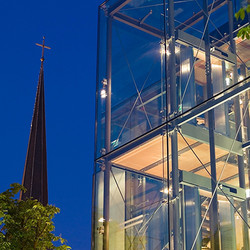R É A M É N A G E M E N T    D E

L ' A S C E N S E U R   D E   L A

S C H A U W E N B U E R G

B E R T R A N G E

2 0 0 5

photos: Romain LambayT U N N E L

C O N C E P T I O N   A R C H I T E C T U R A L E

B E R T R A N G E

2 0 0 2